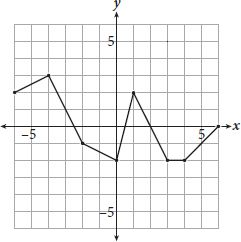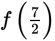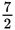# SAT Math Multiple Choice Question 920: Answer and Explanation

### Test Information

Question: 920

4.The complete graph of the function f is shown in the xy plane above. Which of the following is/are equal to –2?

I. f(–1)

II. f(0)

III.• A. I only
• B. II only
• C. II and III only
• D. I, II, and III

Getting to the Answer: Read the graph to find the y-coordinates that correspond to the given x-coordinates (the numbers inside the parentheses) in I, II, and III. The points on the graph of f with x-coordinates -1, 0, and(or 3.5) are (-1, - 1.5), (0,-2), and (3.5, -2). Therefore, of the values given, only 0 (II) and(III) are equal to -2, making (C) correct.